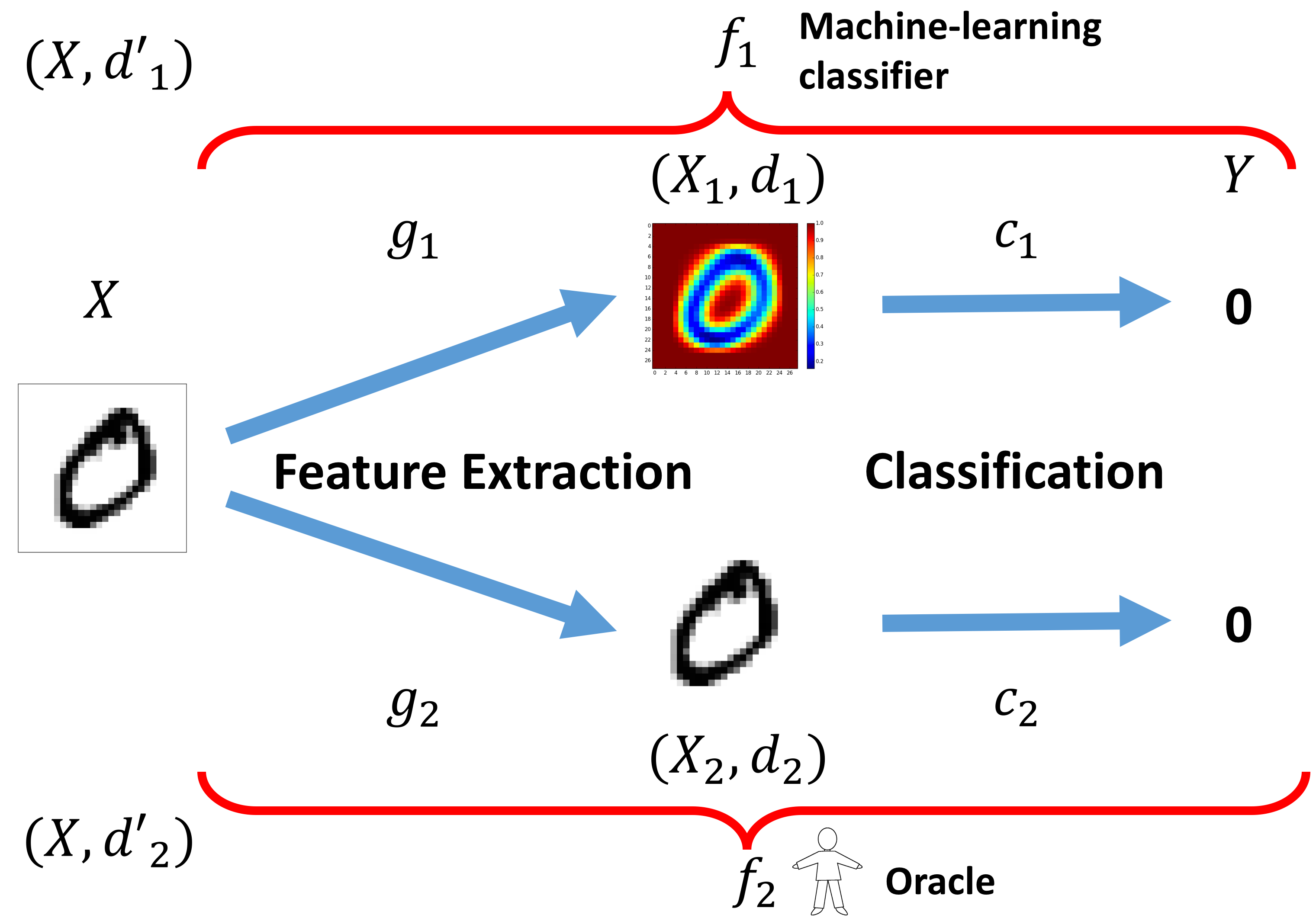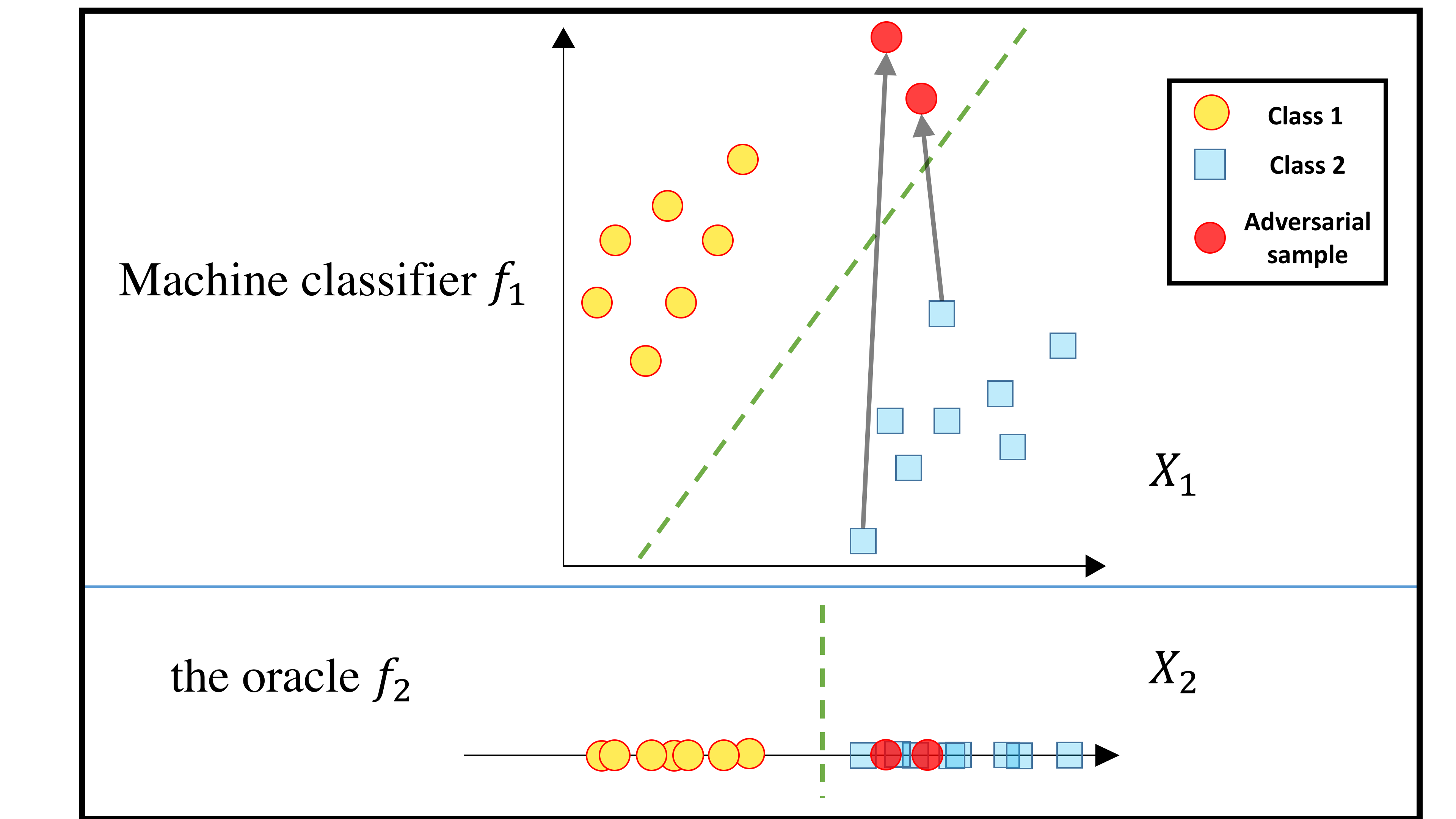# ICLR - A Theoretical Framework for Robustness of (Deep) Classifiers Against Adversarial Samples

### Abstract

Most machine learning classifiers, including deep neural networks, are vulnerable to adversarial examples. Such inputs are typically generated by adding small but purposeful modifications that lead to incorrect outputs while imperceptible to human eyes. The goal of this paper is not to introduce a single method, but to make theoretical steps towards fully understanding adversarial examples. By using concepts from topology, our theoretical analysis brings forth the key reasons why an adversarial example can fool a classifier (f1) and adds its oracle (f2, like human eyes) in such analysis. By investigating the topological relationship between two (pseudo)metric spaces corresponding to predictor f1 and oracle f2, we develop necessary and sufficient conditions that can determine if f1 is always robust (strong-robust) against adversarial examples according to f2. Interestingly our theorems indicate that just one unnecessary feature can make f1 not strong-robust, and the right feature representation learning is the key to getting a classifier that is both accurate and strong-robust.

Recent studies are mostly empirical and provide little understanding of why an adversary can fool machine learning models with adversarial examples. Several important questions have not been answered yet:

• What makes a classifier always robust to adversarial examples?
• Which parts of a classifier influence its robustness against adversarial examples more, compared with the rest?
• What is the relationship between a classifier’s generalization accuracy and its robustness against adversarial examples?
• Why (many) DNN classifiers are not robust against adversarial examples ? How to improve?

This paper uses the following framework

• to understand adversarial examples (by considering the role of oracle):• The following figure provides a simple case illustration explaining unnecessary features make a classifier vulnerable to adversarial examples:• The following figure tries to explain why DNN models are vulnerable to adversarial examples:``````@article{wang2016theoretical,
title={A theoretical framework for robustness of (deep) classifiers under adversarial noise},
author={Wang, Beilun and Gao, Ji and Qi, Yanjun},
journal={arXiv preprint},
year={2016}
}
``````